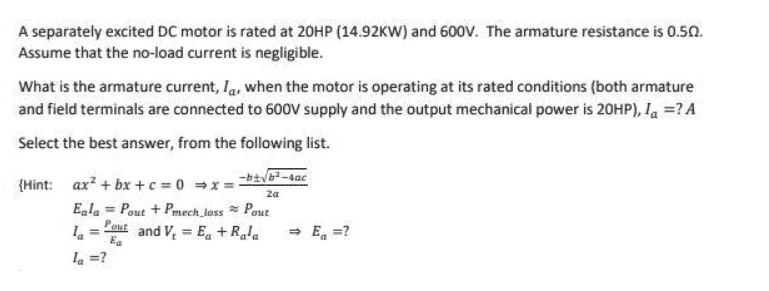# Separately excited DC motor problem (Electrical Engineering)

• Engineering
• Pipsqueakalchemist
Field loss is not relevant in this problem as the motor is separately excited, and you are asked about the output power. The field loss component of the current does not flow through armature and is irrelevant here unless you want to calculate the efficiency (and if the field resistance was given).

#### Pipsqueakalchemist

Homework Statement
I have the image of the question below
Relevant Equations
Hello, so for this question I know how to solve it but I still have a few questions. First when the question means it's rated at 20 HP and 600 V, it's referring to the source voltage and Pin correct? Also in order to solve this question, the rotation loss Prot was neglected. This was because at No load condition the armature current was zero meaning that we were assuming a ideal DC motot hence Prot = 0 correct?Pipsqueakalchemist said:
it's referring to the source voltage and Pin correct?
The 20HP rating denotes output mechanical power (i.e. gross mechanical power developed-mechanical losses)
Pipsqueakalchemist said:
This was because at No load condition the armature current was zero meaning that we were assuming a ideal DC motot hence Prot = 0 correct?
Right.
No load current is neglected, meaning zero armature copper loss and zero rotational loss.

cnh1995 said:
The 20HP rating denotes output mechanical power (i.e. gross mechanical power developed-mechanical losses)

Right.
No load current is neglected, meaning zero armature copper loss and zero rotational loss.
Ok so the rate 20 HP represents Pout correct?
Also in a ideal motor both rotational and armature loss are zero, but what about field loss?
thanks

Pipsqueakalchemist said:
Ok so the rate 20 HP represents Pout correct?
Also in a ideal motor both rotational and armature loss are zero, but what about field loss?
thanks
Field loss is not relevant in this problem as the motor is separately excited, and you are asked about the output power. The field loss component of the current does not flow through armature and is irrelevant here unless you want to calculate the efficiency (and if the field resistance was given).

cnh1995 said:
Right.
No load current is neglected, meaning zero armature copper loss and zero rotational loss
I think this statement is misguiding.
The motor is not "ideal" as there would be copper losses in the armature circuit when there is load on the motor (i.e. current in the armature circuit)
As the no load current is negligible, the motor seems be rotating smoothly and there are no friction/windage losses. This means the rotational losses (i.e. friction/windage) should be zero at any speed and any loading condition.

But there will be some copper loss in the armature circuit due to its resistance.

Only in case of no-load, armature copper loss and rotational loss are both 0. At any other loading condition, rotational loss will be 0, but copper loss will be non-zero.